# Semi-Fredholm operator

Letandbe two Banach spaces and let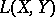denote the Banach space of all continuous (bounded) operators frominto(cf. also Banach space; Continuous operator). For an operatorin, letbe the set of allsuch that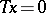and letbe the quotient space, wheredenotes the range of. By definition,is a semi-Fredholm operator if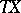is closed (i.e. it is a normally-solvable operator) and at least one of the vector spacesand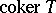is of finite dimension. (The definition is partially redundant, since if the dimension ofis finite,is closed.)

For a semi-Fredholm operator, its index, i.e.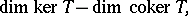is uniquely determined either as an integer, or as plus or minus infinity. In the first caseis a Fredholm operator. Cf. also Index of an operator.

The setof all semi-Fredholm operators inis open inand the index is constant on each connected component of. Moreover, ifis a compact operator inandis in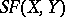, then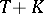is also inand its index equals that of. Due to these properties, semi-Fredholm operators play an important role in linear and non-linear analysis. They were first explicitly considered by I.C. Gohberg and M.G. Krein [a1] and T. Kato [a2], who also treated the case whenis unbounded.

How to Cite This Entry:
Semi-Fredholm operator. C. Foias (originator), Encyclopedia of Mathematics. URL: http://encyclopediaofmath.org/index.php?title=Semi-Fredholm_operator&oldid=12472
This text originally appeared in Encyclopedia of Mathematics - ISBN 1402006098